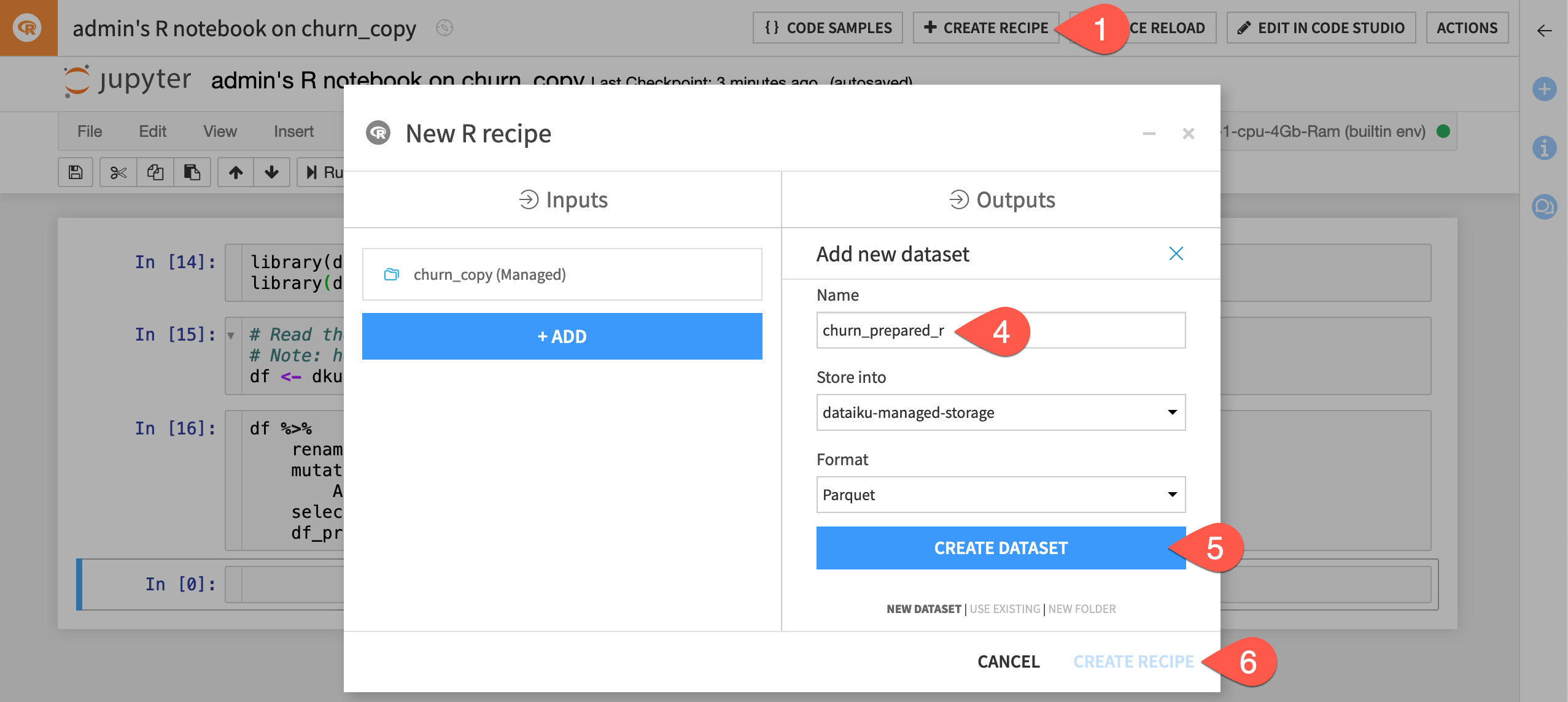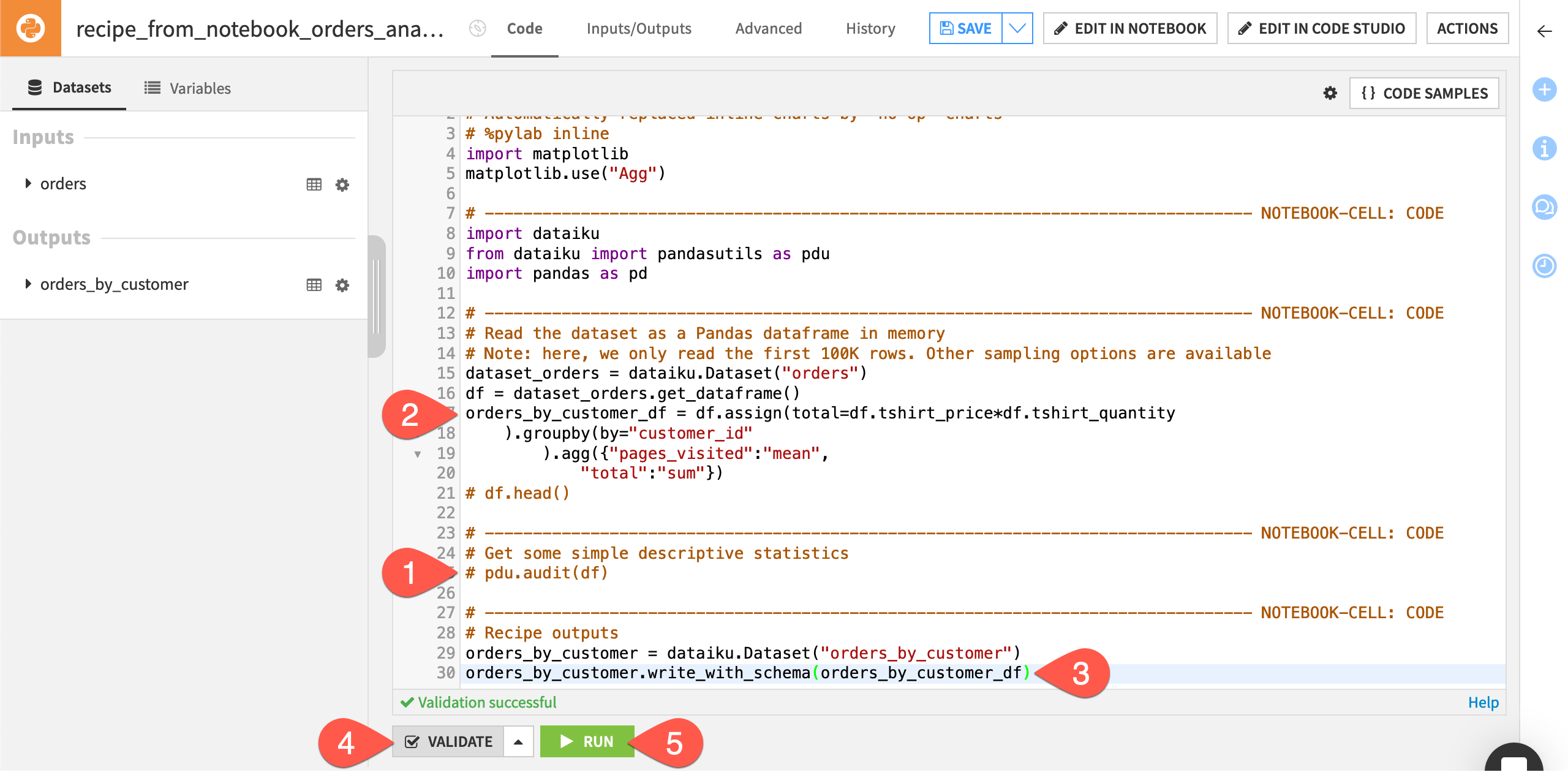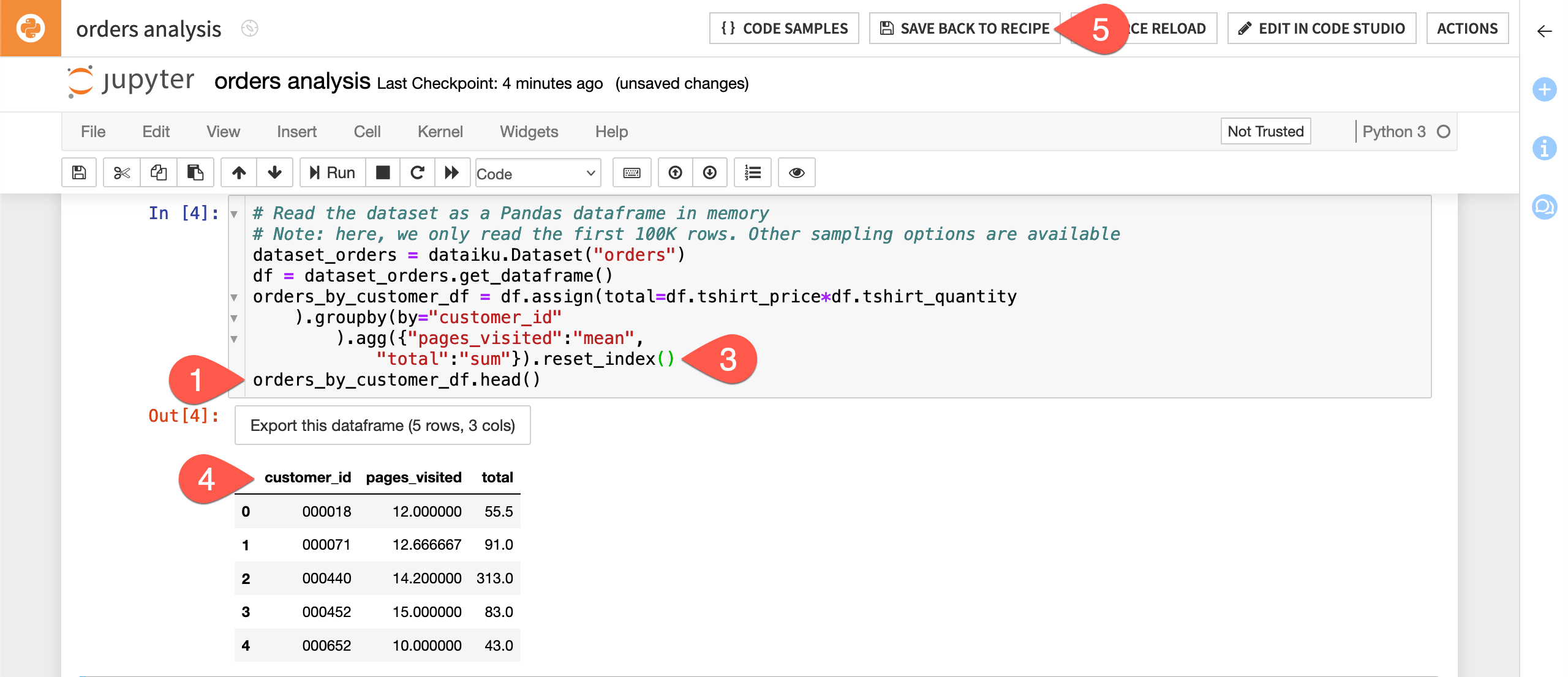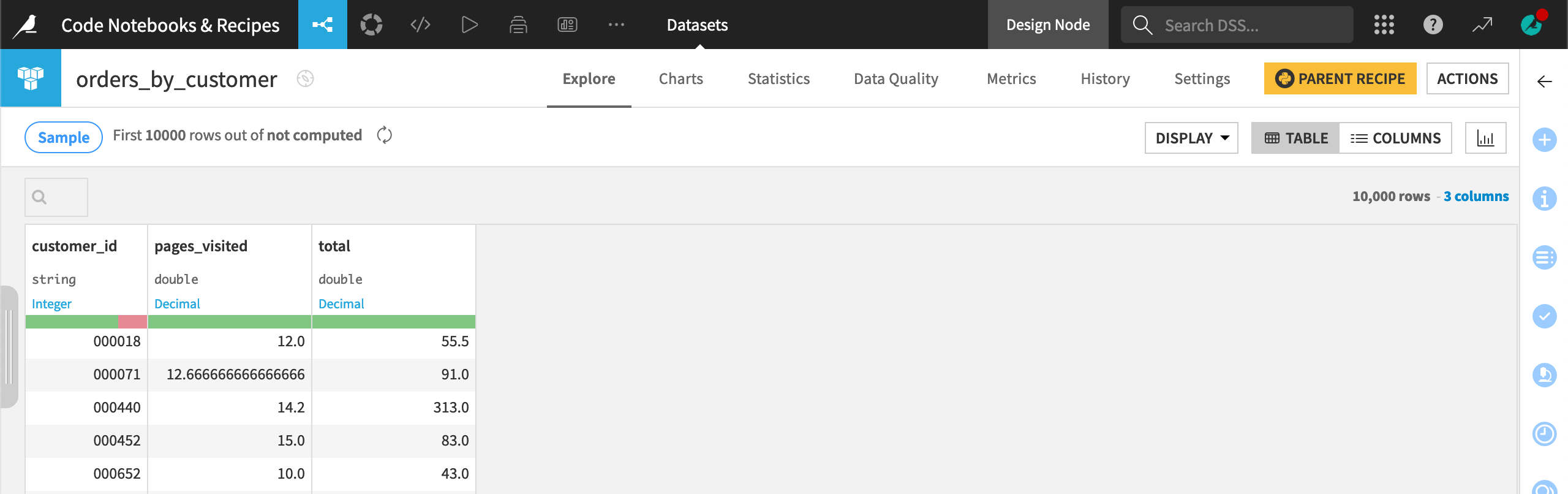# Hands-On Tutorial: Code Recipes¶

In this hands-on tutorial, you will learn how to create, edit, and run Dataiku code recipes, as well as how to navigate back and forth between code recipes and code notebooks.

Note

This hands-on tutorial only covers the use of Python recipes in Dataiku, but the logic is similar for all code recipes. To learn more about using R recipes and, more broadly, R in Dataiku, follow this Academy course.

## Let’s Get Started!¶

You will work with a sample project containing data from the fictional Haiku T-Shirt company.

### Prerequisites¶

• Some familiarity with the basics of Dataiku (we recommend having completed the Basics courses);

• Some familiarity with coding in Python and using Jupyter notebooks.

### Technical Requirements¶

• An instance of Dataiku - version 8.0 or above (Dataiku Online can also be used).

## Create Your Project¶

You can get started in one of the following ways:

### Create a New Project¶

From the homepage, click +New Project > DSS Tutorials > Developer > Code Recipes (Tutorial).

Note

You can also download the starter project from this website and import it as a zip file.

### Continue in the Previous Hands-On Tutorial¶

If you are following the Code in Dataiku DSS course and have already completed the previous tutorial on Code Notebooks, you can continue working in the same Dataiku project.## Create a Recipe from a Notebook¶

Code recipes can be created from the Flow, but you can also create them from existing notebooks. This can be particularly useful for deploying exploratory work from notebooks to the Flow.

In this exercise, we will create a Python code recipe from the Jupyter notebook that was created in the Code Notebooks hands-on.

• Navigate to the Notebooks page and open the orders analysis Python notebook.

• From within the notebook, click Create Recipe > Python recipe.• Select the orders dataset as the input (since that is the dataset used in the notebook).

• Create a new output dataset and name it orders_by_customer.

• Click Create Recipe.

## Edit Code in a Recipe¶

In the resulting recipe, all the code from the Jupyter notebook has been transferred to the recipe code editor. Notice that Dataiku has added a number of commented out lines, each of which shows the beginning of a notebook cell. This way, if we need to edit the recipe in a notebook again, our existing cells are maintained.

The editor has also added two lines for the recipe output based on the name of the output dataset we created in the recipe dialog. We’ll discuss this below.We now want to use the code from the notebook as a basis to create a simple group by function and group orders by customer, aggregating their past interactions. In the Basics courses, we accomplished this with a visual Group recipe, but it can also be easily accomplished with Python code.

Before adding the relevant code, let’s comment out the following lines, which served for displaying insights in the notebook, but are no longer useful when working with a recipe.

• Comment out the following lines of code:

• df.head(); and

• pdu.audit(df)

Next, we will provide the code that aggregates the orders by customer.

• In a new line right below df = dataset_orders.get_dataframe(), enter the following code:

orders_by_customer_df = df.assign(total=df.tshirt_price*df.tshirt_quantity
).groupby(by="customer_id"
).agg({"pages_visited":"mean",
"total":"sum"})This creates a new dataframe with rows grouped by customer_id. For each customer, we’ve computed the average number of pages on the Haiku T-shirt website visited by the customer during orders, and the sum total of the value of orders made by the customer, where the value of each order is the price of each t-shirt multiplied by the number of t-shirts purchased.

Finally, Dataiku has added lines for the recipe output. However, it cannot know which dataframe (df or orders_by_customer_df) we want to output as the orders_by_customer dataset. Accordingly:

• In the last line of code, change “pandas_dataframe” to orders_by_customer_df.

• Click “Validate” to check the validity of the code. It should display “Validation successful”.

• Run the recipe.• When it completes, explore the output dataset.

Notice that the output dataset does not contain the customer_id column, even though this was the key we grouped by. We’d like to have it for reference.### Edit the Contents of a Code Recipe in a Code Notebook¶

• To diagnose the issue, reopen the Python recipe (clicking Parent Recipe is one option), then click Edit in Notebook.

Tip

Although it’s not the case in this tutorial, often when editing the contents of a code recipe in a Jupyter notebook, the code will appear in one single cell. You can use the Ctrl + Shift + - shortcut to split code into multiple cells.

This opens a Jupyter notebook with the recipe code, where we can interactively test it.

• Uncomment the df.head() function and change df to orders_by_customer_df, so that the new function is as follows:

orders_by_customer_df.head()

• Run the first three cells.

The output shows that the orders_by_customer_df dataframe has the customer_id information; however, the dataframe has a hierarchical index.• In order to flatten the index, add .reset_index() to the code that defines the dataframe so that it looks like the following:

orders_by_customer_df = df.assign(total=df.tshirt_price*df.tshirt_quantity
).groupby(by="customer_id"
).agg({"pages_visited":"mean",
"total":"sum"}).reset_index()

• Re-run the third cell to see how the dataframe has changed.• Click Save back to recipe.

• Comment out orders_by_customer_df.head(), validate, and run the recipe again.

Now the output dataset contains a customer_id column.## What’s Next?¶

To go further with using Python recipes in Dataiku:

If you want to learn more about using other code recipes, you might want to check out: